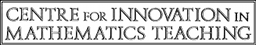Multiple Choice Questions
Unit A4
Ratio and Proportion
For each of these questions, choose the option (A, B, C or D) that is TRUE.
1
Divide £200 in the ratio 3 : 2. What is the result?
• (A)
£140 : £60
• (B)
£80 : £120
• (C)
£300 : £200
• (D)
£120 : £80
2
The ratio of men to women at a party was 6 : 7, respectively.

There were 28 women. Calculate the number of people at the party.

• (A)
24
• (B)
28
• (C)
52
• (D)
104
3
A sum of £300 is divided in the ratio 2 : 3.

Calculate the amount of the LARGER share.

• (A)
£120
• (B)
£150
• (C)
£180
• (D)
£240
4
A metal is made from copper, zinc and lead in the ratio 13 : 6 : 1. The mass of the zinc is 90 kg.

Calculate the mass of the metal.

• (A)
195 kg
• (B)
210 kg
• (C)
285 kg
• (D)
300 kg
5
£x is divided among three boys, Ryan, Keith and Andrew, in the ratio 2 : 3 : 7, respectively.

If Andrew gets £15 more than Keith, what is the value of x ?

• (A)
£27
• (B)
£45
• (C)
£57
• (D)
£180
Total: - of marks